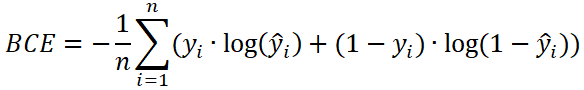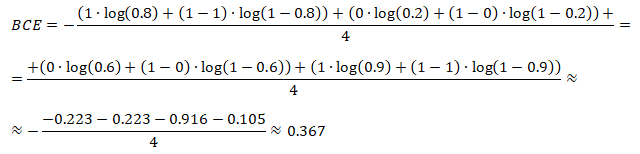# Calculate Binary Cross-Entropy using TensorFlow 2

Binary cross-entropy (BCE) is a loss function that is used to solve binary classification problems (when there are only two classes). BCE is the measure of how far away from the actual label (0 or 1) the prediction is.

The formula to calculate the BCE:• `n` - the number of data points.
• `y` - the actual label of the data point. Also known as true label. Can only be 0 or 1.
• `ŷ` - the predicted probability of the data point. Between 0 and 1. This value is returned by model.

Let's say we have the following sets of numbers:

Here is example how BCE can be calculated using these numbers:TensorFlow 2 allows to calculate the BCE. It can be done by using `BinaryCrossentropy` class.

``````from tensorflow import keras

yActual = [1, 0, 0, 1]
yPredicted = [0.8, 0.2, 0.6, 0.9]

bceObject = keras.losses.BinaryCrossentropy()
bceTensor = bceObject(yActual, yPredicted)
bce = bceTensor.numpy()

print(bce)``````

BCE also can be calculated by using `binary_crossentropy` function.

``````bceTensor = keras.losses.binary_crossentropy(yActual, yPredicted)
bce = bceTensor.numpy()``````